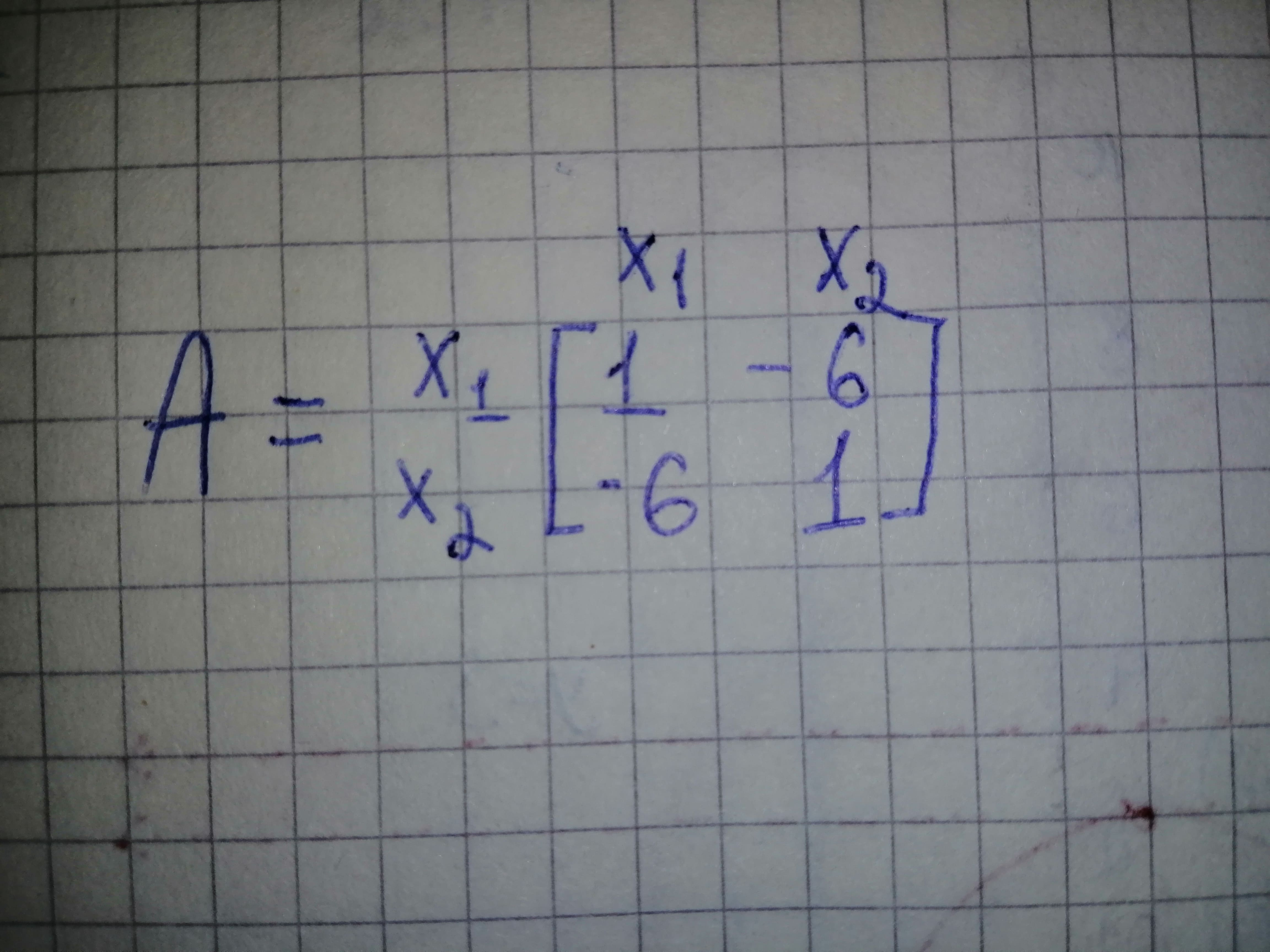# Find out what kind of conic section the following quadratic form represents and transfoRamsey 2020-12-05 Answered

Find out what kind of conic section the following quadratic form represents and transform it to principal axes. Express ${\stackrel{\to }{x}}^{T}=\left[{x}_{1}{x}_{2}\right]$ in terms of the new coordinate vector ${\stackrel{\to }{y}}^{T}=\left[{y}_{1}{y}_{2}\right]$
${x}_{1}^{2}-12{x}_{1}{x}_{2}+{x}_{2}^{2}=70$

You can still ask an expert for help

• Questions are typically answered in as fast as 30 minutes

Solve your problem for the price of one coffee

• Math expert for every subject
• Pay only if we can solve itottcomn

Given quadratic equation is ${x}_{1}^{2}-12{x}_{1}{x}_{2}+{x}_{2}^{2}=70$
The matrix representation of quadratic form isNow trace $\left(A\right)=2\phantom{\rule{1em}{0ex}}\text{and}\phantom{\rule{1em}{0ex}}det\left(A\right)=-35$
Characteristic equation of matrix A is ${\lambda }^{2}-2\lambda -35=0$
Therefore eigen values of matrix A are $\lambda =-5,7$
Since matrix A has both positive and negative eigen values, therefore matrix A is indefinite.